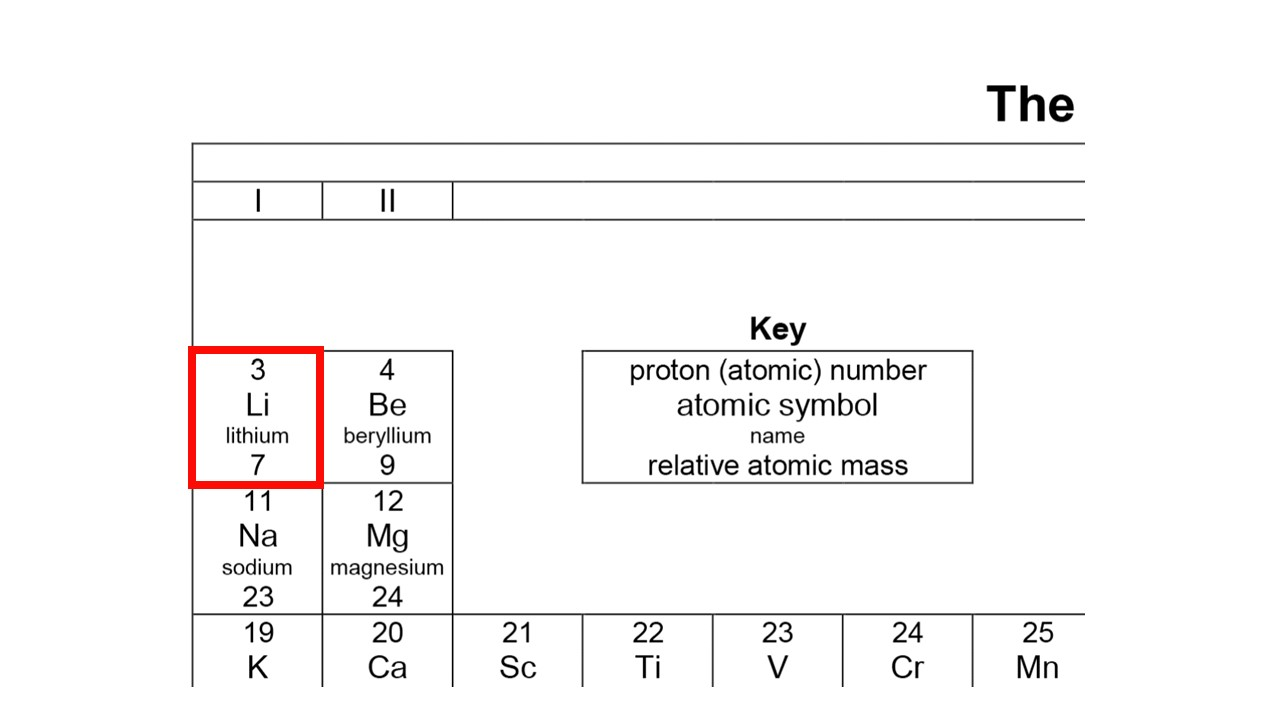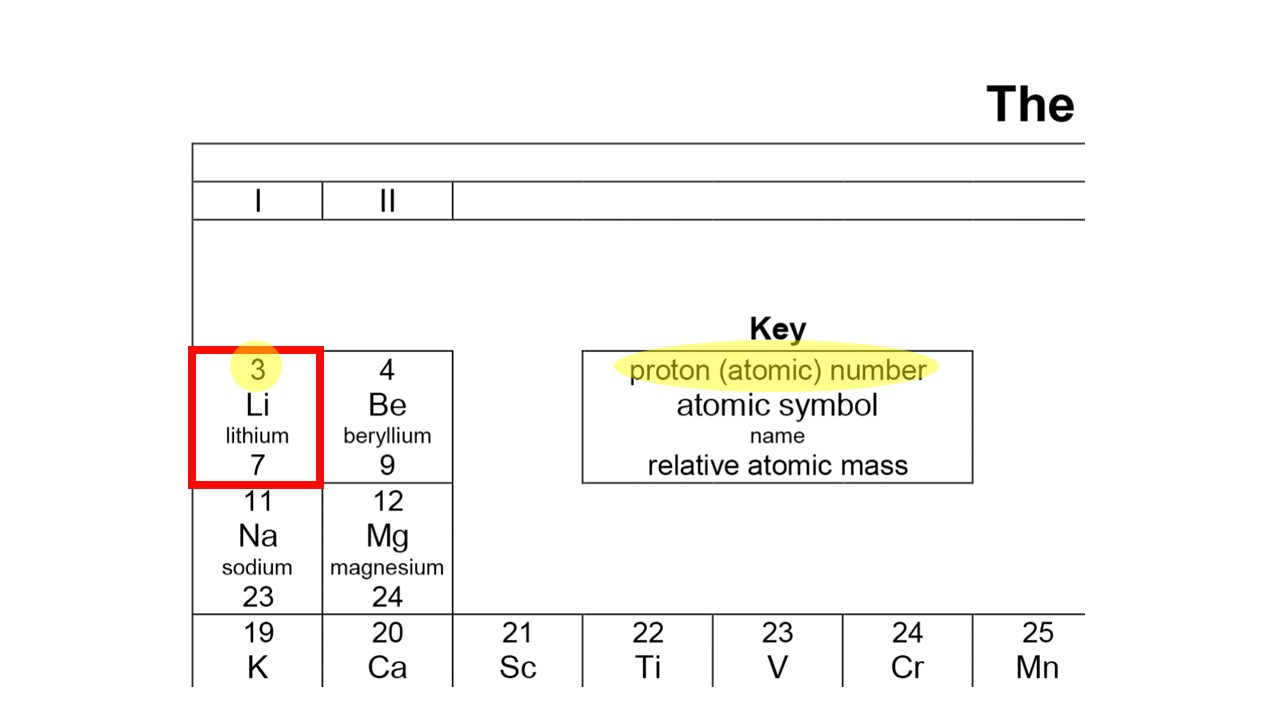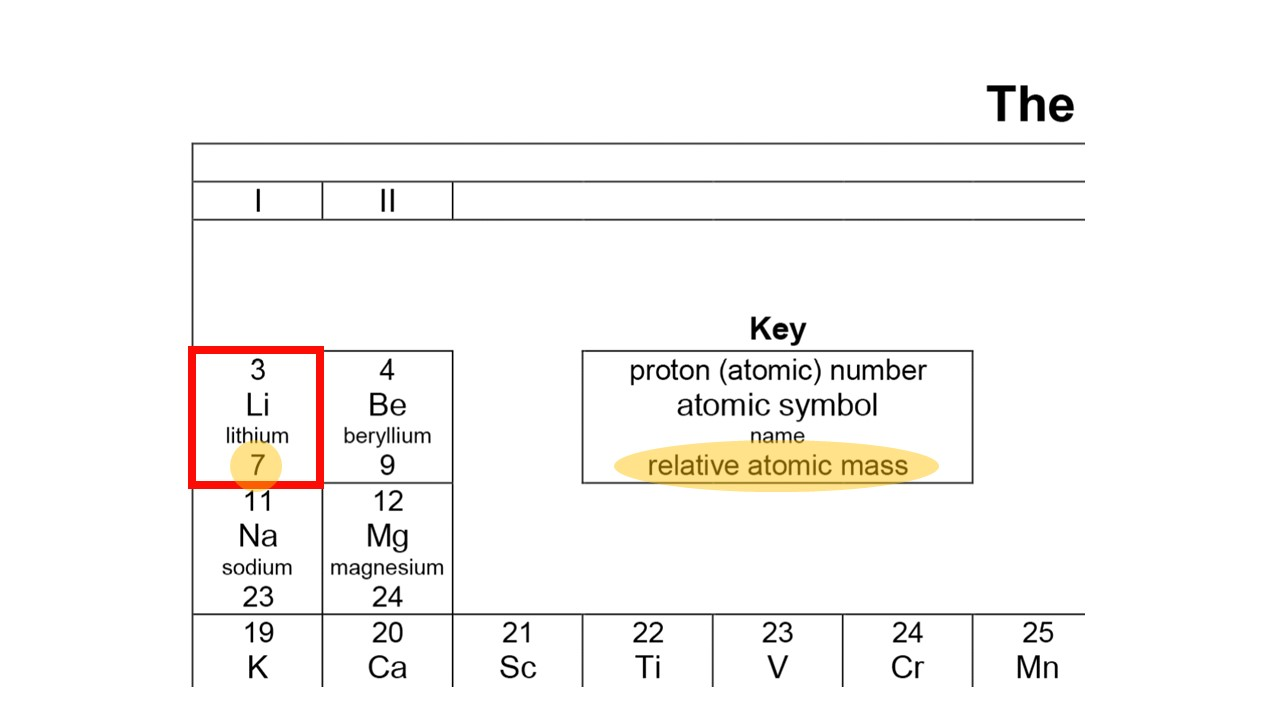## 1. An atom has more space than structureAn atom of lithium has a dense nucleus of protons and neutrons at the centre, surrounded by electrons moving in the vast space

The word ‘structure’ reminds us of something concrete, like a building. But in terms of volume, an atom is mostly space. In the centre, the nucleus that comprises protons and neutrons takes up just 0.001% of the volume. The remainder is a big empty void in which electrons wander around.

## 2. Atomic structure is the arrangement of subatomic particles

Atomic structure does not refer to the concrete, detailed layout of an atom.

Instead, it simply refers to the number and arrangement of subatomic particles: protons, neutrons, and electrons.

## 3. May I have your number?

There are two very important numbers that give us the number of subatomic particles in an atom:

• Proton number gives the number of protons. Because an atom is electrically neutral, the proton number also equals the number of electrons.
• Mass number gives the total number of protons and neutrons in the nucleus.

We usually write these numbers on the left of the chemical symbol, with the mass number on top and the proton number below. For example, the notation above shows that lithium has a mass number of 7 and a proton number of 3.

## 4. Uncovering the number of neutrons with quick maths!

The difference between the mass number and the proton number is the number of neutrons.

So to find the number of neutrons in the nucleus of a lithium atom, we simply compute 7 − 3 = 4 neutrons.

## 5. Stalk the Periodic Table for the atomic and mass numbers

•Find lithium on the Periodic Table by looking out for its symbol Li
•The proton number is the smaller number at the top of the tile
•The mass number is the larger number at the bottom of the tile

The proton and mass numbers can also be found on the Periodic Table. But unlike the notation that we just learned, the Periodic Table places the proton number on top. Confusing?

The trick is, with the exception of hydrogen, the proton number is the smaller number.

## 6. Electrons are further arranged into shellsWe draw the electrons shells in a simplified way, as circles. The electrons in a shell are then drawn as dots on top of the circle.

Electrons do not just move randomly. Some electrons are found closer to the nucleus, while others are further away.

We can think of electrons as being arranged in electron shell. The first shell is the smallest shell closest to the nucleus. In the first shell, the electrons are attracted strongly by the positive nucleus, hence they have the least energy.

The second shell is further away form the nucleus, containing electrons with more energy.

## 7. Electrons are the true safe distancing ambassadors

Electrons like to be close to the nucleus, but they do not like to crowd out the shell. The first shell is the smallest, holding up to 2 electrons.

Take lithium with 3 electrons as an example. Only 2 of its 3 electrons can occupy the first shell. Therefore, the remaining electron will be found in the second shell. For lithium, the second shell is the outermost shell.

We sometimes also call the outermost shell the valence shell.